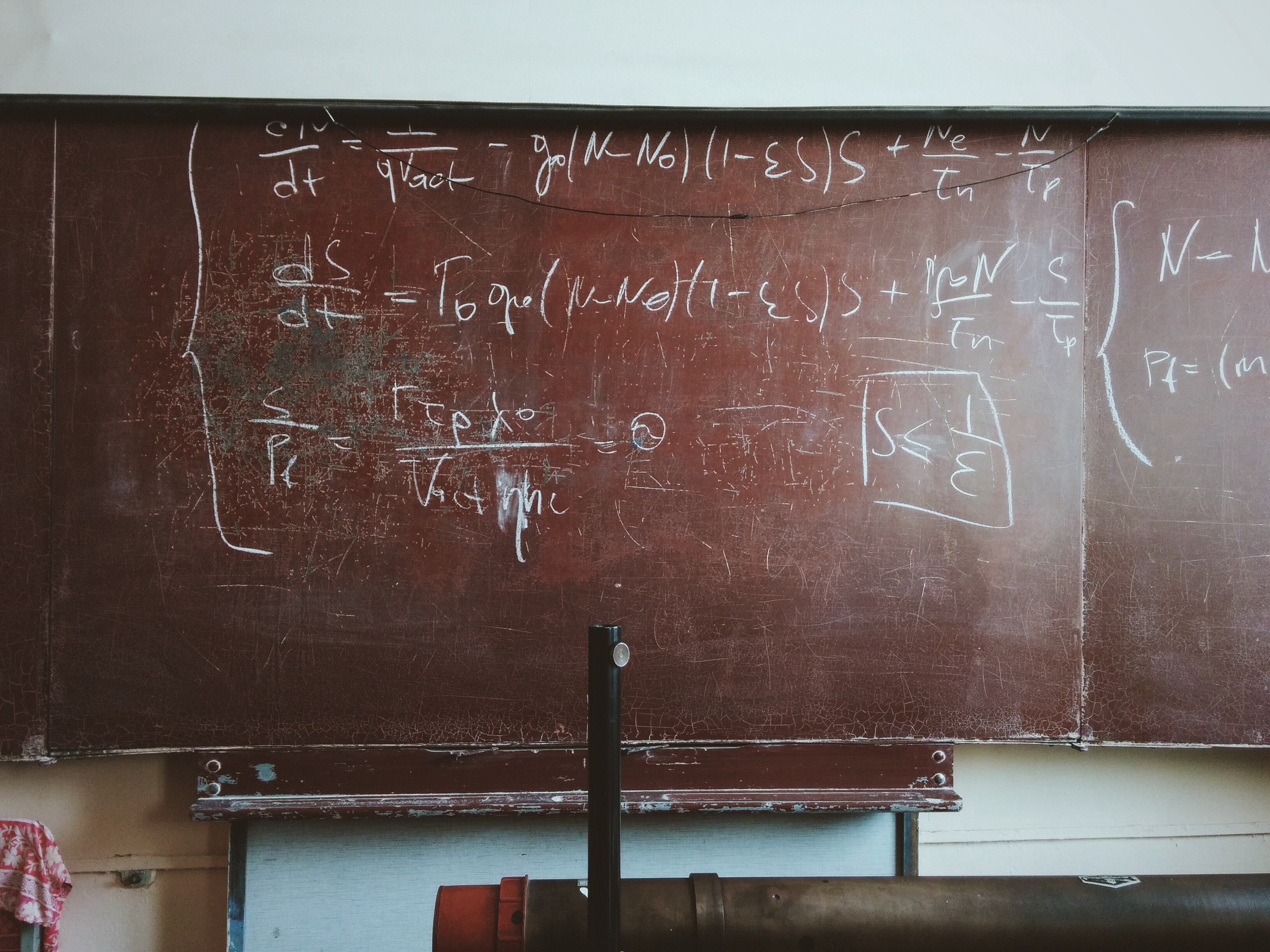# the backpropagation equations for a 3 layer neural net

Written onIn this post we'll derive the backpropagation equations for a three layer neural net that has an arbitrary number of nodes in each layer; the results will be applicable, e.g., to the neural net we used earlier to classify handwritten numbers. For simplicity we'll set all the biases to zero. We'll also be applying tanh as the activation function for the hidden layer and softmax for the output layer.

We'll represent the numerical values held in each layer by row vectors $$l^{0}$$, $$l^{1}$$, and $$l^{2}$$, where superscript $$0$$ represents the input layer, $$1$$ represents the hidden layer, and $$2$$ represents the output layer.

Then the error, $$\epsilon$$, is proportional to

$$\frac{1}{2}\sum_k \left( l^2_k - y_k \right)^2,$$

where $$y_k$$ is the true label corresponding to the $$k^{th}$$ neuron in the output layer; we have included the factor of $$1/2$$ so that the equations that follow will be a bit cleaner. (Besides, we are eventually going to re-scale by a hyper-parameter $$\alpha$$ between $$0$$ and $$1$$ anyway.) The value of the $$l^{th}$$ neuron in the first layer is given by

$$l^1_l = t_l\left[ \sum_m l_m^0 w_{ml}^{01} \right],$$

where $$t_l$$ is the tanh function evaluated at the $$l^{th}$$ neuron, and $$w^{01}_{ml}$$ is the weight from the $$m^{th}$$ neuron of layer $$0$$ to the $$l^{th}$$ neuron of layer $$1$$. The above equation corresponds to the matrix equation

$$\textbf{l}^1=\tanh(\textbf{l}^0\textbf{w}^{01}).$$

Similarly, the value of the $$n^{th}$$ neuron in the second layer is given by

$$l_n^2 = s_n\left[\sum_p l_p^1 w_{pn}^{12}\right],$$

where $$s_n$$ is the softmax function evaluated at the $$n^{th}$$ neuron. For the components of the gradient corresponding to $$\textbf{w}^{12}$$ we therefore have

$$\frac{ \partial \epsilon }{ \partial w^{12}_{qr} } = \frac{1}{2} \sum_k \frac{ \partial }{ \partial w^{12}_{qr} }\left(l_k^2-y_k \right)^2 = \sum_k \left(l_k^2-y_k\right)\frac{\partial l_k^2}{\partial w_{qr}^{12}}=$$
$$\sum_k \left(l_k^2-y_k\right)\frac{\partial}{\partial w_{qr}^{12}}\left[s_k\left(\sum_p l_p^1 w_{pk}^{12}\right)\right]=\sum_{k,p}\left(l_k^2-y_k\right)s_k' l_p^1\frac{\partial w_{pk}^{12}}{\partial w_{qr}^{12}}=$$
$$\sum_{k,p}\left(l_k^2-y_k\right) s_k' l_p^1\delta_{pq}\delta_{kr}=\left(l_r^2-y_r\right) s_r' l_q^1.$$

Hence, we have

$$\frac{ \partial \epsilon }{ \partial w^{12}_{qr} }=\left(l_r^2-y_r\right) s_r' l_q^1.$$

For the components of the gradient corresponding to the weights in the hidden layer we have

$$\frac{\partial \epsilon}{\partial w_{qr}^{01}}=\sum_k \left(l_k^2-y_k \right)\frac{\partial l_k^2}{\partial w_{qr}^{01}}=\sum_{k,p}\left(l_k^2-y_k\right)\frac{\partial l_k^2}{\partial l_p^1}\frac{\partial l_p^1}{\partial w_{qr}^{01}}=$$
$$\sum_{k,p}\left(l_k^2-y_k\right)\frac{\partial l_k^2}{\partial l_p^1}t_p'\sum_m l_m^0 \frac{\partial w_{mp}^{01}}{\partial w_{qr}^{01}}=$$
$$\sum_{k,p,m}\left(l_k^2-y_k\right)\frac{\partial}{\partial l_p^1}\left[s_k\left(\sum_s l_s^1 w_{sk}^{12}\right)\right]t_p' l_m^0 \delta_{mq}\delta_{pr}=$$
$$\sum_{k,p,m,s}\left(l_k^2-y_k\right) s_k' w_{sk}^{12}\delta_{ps}t_p'l_m^0 \delta_{mq}\delta_{pr}=\sum_k \left(l_k^2-y_k\right) s_k' w_{rk}^{12}t_r' l_q^0.$$

In summary, for the components of the gradient we have

$$\frac{\partial \epsilon}{\partial w_{qr}^{01}}=\sum_k \left(l_k^2-y_k\right) s_k' w_{rk}^{12}t_r' l_q^0.$$

and

$$\frac{ \partial \epsilon }{ \partial w^{12}_{qr} }=\left(l_r^2-y_r\right) s_r' l_q^1.$$

We can put these equations into a more transparent form by defining $$\textbf{D}_{t'}$$ as a diagonal matrix with the $$t_j'$$ values along the diagonal and a similar matrix $$\textbf{D}_{s'}$$ for the values $$s_j'$$. With these definitions we have

$$\frac{\partial \epsilon}{\partial w_{qr}^{12}}=\left[\textbf{l}^1\otimes\left(\textbf{l}^2-\textbf{y}\right) \textbf{D}_{s'} \right]_{qr},$$

and

$$\frac{\partial \epsilon}{\partial w_{qr}^{01}}=\{ \textbf{l}^0 \otimes \left[ \left( \textbf{l}^2-\textbf{y} \right) \textbf{D}_{s'} \textbf{w}^{12,T} \textbf{D}_{t'} \right] \}_{qr}$$

where $$\otimes$$ is the outer product. Introducing a re-scaling hyperparameter $$\alpha$$, the corrections to the weights (denoted by asterisks) are then

$$w_{qr}^{12*}=w_{qr}^{12}-\alpha \left[\textbf{l}^1\otimes\left(\textbf{l}^2-\textbf{y}\right) \textbf{D}_{s'} \right]_{qr},$$

and

$$w_{qr}^{01*}=w_{qr}^{01}-\alpha \{ \textbf{l}^0 \otimes \left[ \left( \textbf{l}^2-\textbf{y} \right) \textbf{D}_{s'} \textbf{w}^{12,T} \textbf{D}_{t'} \right] \}_{qr}.$$